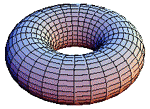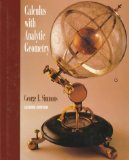Fall 2018 & IAP 2019
Single & Multivariable Calculus
Calculus I and II
Math 18.01A-18.02A, Concourse - MIT

Lectures and Recitations by:
Robert Winters
rwinters@mit.edu

Office: 16-137
Phone: x3-2050
(but e-mail is better)

 Calendar of topics and homework assignments Solutions (username/password required)

Concourse 18.01A (CC.181a)
Stellar site

Concourse 18.02A (CC.182a)
Stellar siteThe text for the course is
Calculus with Analytic Geometry, 2nd Edition by George F. Simmons (ISBN 9780070576421), published by McGraw-Hill
[Click on the image below for prices.]You can probably get the best price for this book from MIT students who took this course. The book has not changed in years.

In addition, you will want to purchase or download a copy of the 18.02A Course Notes.

• Ch. 1 - Numbers, Functions, and Graphs

• Ch. 2 - The Derivative of a Function

• Ch. 3 - Computation of Derivatives

• Ch. 4 - Applications of Derivatives

• Ch. 5 - Indefinite Integrals and Differential Equations

• Ch. 6 - Definite Integrals

• Ch. 7 - Applications of Integration

• Ch. 8 - Exponential and Logarithm Functions

• Ch. 9 - Trigonometric Functions

18.01A Topics

Ch. 10 - Methods of Integration

Ch. 11 - Further Applications of Integration

Ch. 12 - Indeterminate Forms and Improper Integrals

Ch. 13 - Infinite Series of Constants

Ch. 14 - Power Series

• Ch. 15 - Conic Sections

• Ch. 16 - Polar Coordinates

18.02A Topics

• Ch. 17 - Parametric Equations, Vectors in the Plane

• Ch. 18 - Vectors in Three-Dimensional Space, Surfaces

• Ch. 19 - Partial Derivatives

• Ch. 20 - Multiple Integrals

• Ch. 21 - Line and Surface Integrals, Green's Theorem, Gauss' Theorem, and Stokes' Theorem

Announcements:

Thanks for attending the course.

 Approximate letter grades for Exam #1 (18.01A) Total points on exam was 50. Median score was 34.5. Mean score was 37.3. Standard deviation was 6.9. score grade score grade 45+ A 29+ C+ 42+ A– 27+ C 38+ B+ 25+ C– 35+ B 23+ D 32+ B– 0-22 F

 Approximate letter grades for Exam #2 (18.01A Final) Total points on exam was 76. Median score was 42.5. Mean score was 44.3. Standard deviation was 4.8. score grade score grade 67+ A 47+ C+ 63+ A– 43+ C 59+ B+ 39+ C– 55+ B 37+ D 51+ B– 0-36 F
 Approximate letter grades for Exam #3 (18.02A) Total points on exam was 60. Median score was 38. Mean score was 36.8. Standard deviation was 10.1. score grade score grade 53+ A 38+ C+ 50+ A– 35+ C 47+ B+ 32+ C– 44+ B 30+ D 41+ B– 0-29 F

 Approximate letter grades for Exam #4 (18.02A) Total points on exam was 50. Median score was 40.5. Mean score was 39.5. Standard deviation was 10.5. score grade score grade 45+ A 30+ C+ 42+ A– 27+ C 39+ B+ 25+ C– 36+ B 23+ D 33+ B– 0-22 F
 Approximate letter grades for Exam #5 (18.02A) Total points on exam was 50. Median score was 22.5. Mean score was 26.3. Standard deviation was 11.9. score grade score grade 45+ A 30+ C+ 42+ A– 27+ C 39+ B+ 25+ C– 36+ B 23+ D 33+ B– 0-22 F

 Approximate letter grades for Final Exam (18.02A) Total points on exam was 90. Median score was 45. Mean score was 45.8. Standard deviation was 18.0. score grade score grade 82+ A 57+ C+ 77+ A– 52+ C 72+ B+ 47+ C– 67+ B 43+ D 62+ B– 0-42 F

This sequence, intended for students who have had a full year of high school calculus, begins with 18.01A, a six-week review of one-variable calculus, emphasizing integration techniques and applications, polar coordinates, improper integrals, sequences, and infinite series. Prerequisite is a score of 4 or 5 on the Advanced Placement Calculus AB exam or a passing grade on the first half of the 18.01 Advanced Standing Exam, covering differentiation and elementary integration. Most students completing 18.01A continue directly into 18.02A, in which the remaining weeks of the fall term is devoted to the material in the first half of Calculus II. 18.02A is taught at the same pace as 18.02. Concourse students complete the second half of Calculus II during Independent Activities Period (IAP) in January.

Supplementary Notes:
18.01 Supplementary Notes authored by Prof. Arthur Mattuck of the MIT Mathematics Department, exercises by David Jerison.
18.02 Supplementary Notes authored by Prof. Arthur Mattuck of the MIT Mathematics Department.

Text: Calculus with Analytic Geometry, 2nd Edition by George F. Simmons (ISBN 9780070576421), published by McGraw-Hill

18.01/02 Mathlets (to appear)

Homework: Homework will be posted on the course website and will be due approximately weekly. Typical assignments will include some exercises that are to be turned in as well as additional practice problems. Homework may be submitted in class or at my office, but it should be completed by the posted due date. Additional time will only be given if requested before the due date and if appropriate for the circumstances. You should not consult any solutions manual in preparing your assignments. You are encouraged to work with your fellow students on the homework, but your written solutions must be your own. Solutions will be made available (as PDF files) on the course website shortly after they are due.

Class during IAP is expected to be at the same time as the mainstream class: Daily, Mon-Fri, 12:00-1:00pm. We could meet for longer on some days in order to create a free day, but only if everyone agrees.

We'll also schedule a few recitation times during the week based on the preferences of the class. Alternatively, we can hold informal meetings on several afternoons around the conference table in the Concourse Lounge.

 Condensed Syllabus: (See the Calendar for day-by-day details and assignments, updated as the course proceeds.) 18.01A Topics: Review of basic ideas of Differential Calculus. (Chaps. 2-7) Applications of Integration: area, volume, volume of solids of revolution, arclength, area of a surface of revolution, work and energy, hydrostatic force. (Chap. 7) Techniques of integration: substitution, trigonometric integrals, trigonometric substitutions, partial fractions, integration by parts, miscellaneous methods, numerical integration and Simpson's Rule. (Chap. 10) Further Applications of Integration: Center of mass, centroids, moment of inertia. (Chap. 11) Indeterminate forms, L'Hôpital's Rule, improper integrals. Sequences, infinite series, convergent vs. divergent series, comparison tests, integral test, ratio and root tests, alternating series, absolute vs. conditional convergence. (Chap. 13) Power series, interval of convergence, differentiation and integration of power series, Taylor Series and Taylor's Formula, applications to differential equations. (Chap. 14) Probability. (Supplementary Notes) 18.02A Topics: Coordinates, vectors and vector algebra in R2 and R3; dot product, cross product, projection, equations of lines and planes, matrix methods. (Chaps. 17-18 and Notes) Parametric equations of curves in R2 and R3; coordinates, derivatives of vector-valued functions, velocity and acceleration, tangent vectors, arclength; curvature and unit normal vector, tangential and normal components of acceleration, Kepler's Laws and Newton's Law of Gravitation. (Chaps. 17-18) Cylinders and surfaces of revolution, cylindrical and spherical coordinates; parameterized surfaces in R2 and R3. (Chap. 18) Functions of several variables - limits, continuity, and differentiabilty; partial derivatives, gradients, linear approximation, directional derivatives, Chain Rule. (Chap. 19) Optimization - unconstrained and constrained; implicit functions and implicit differentiation. (Chap. 19) Multiple integrals, integration over regions in R2 and R3 and their applications using Cartesian, polar, cylindrical, and spherical coordinates, gravitational attraction. (Chap. 20) Vector fields and their applications. (Notes) Integration over curves in R2 and R3 by parameterization; work integrals, and applications; independence of path and conservative vector fields; Green's Theorem. (Chap. 21) Integration over surfaces in R3 by parameterization - flux integrals, surface area, and applications. (Chap. 21) Calculus of vector fields; curl and divergence of vector fields; Stokes’ Theorem, Divergence Theorem; Maxwell's equations. (Chap. 21)

Topics and Assignments are posted in the Course Calendar.

Singular Sensations - Steve Strogatz in the New York Times

If ever the MIT mail servers are not accessible from the outside world and you need to get in touch, you can also contact me at either robert@math.rwinters.com or Robert@rwinters.com.